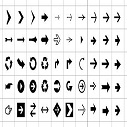# Python Generator Expressions

Generator comprehensions are similar to the list/set comprehensions, the only difference is that we use circular brackets in a generator comprehension. The motive behind the introduction of a generator comprehension in Python is to have a memory-efficient approach in place.

In a generator comprehension, memory is allotted on the go and not on the startup. In the list/set comprehensions, we generate the entire elements first and keep them in memory. However, a generator comprehension employs a lazy-loading approach.

A generator is a function with a yield statement instead of returning a value. The peculiarity of a yield statement is that we can pause the method at any time and resume it back at a later point if needed.

Generator Expressions are somewhat similar to list comprehensions, but the Generator Expressions doesn't construct list object. Instead of creating a list and keeping the whole sequence in the memory, the generator generates the next element in demand.

When a normal function with a return statement is called, it terminates whenever it gets a return statement. But a function with a yield statement saves the state of the function and can be picked up from the same state, next time the function is called.

The Generator Expression allows us to create a generator without the yield keyword.

### Syntax Difference:

Parenthesis are used in Generator Expressions and square brackets are used in list.

```>>> # List Comprehension
>>> list1=[i for i in range(10)]
>>> list1
[0, 1, 2, 3, 4, 5, 6, 7, 8, 9]
>>>

>>> # Generator Expression
>>> gen=(i for i in range(10))
>>> gen
<generator object <genexpr> at 0x000001F8E16C77C8>
>>>
>>> # to print the values from generator, we can loop through it
>>> gen=(i for i in range(10))
>>> for i in gen:
print(i,end=" ")

0 1 2 3 4 5 6 7 8 9
>>>
```

### Difference between Generator Expressions and List Comprehensions

The generator yields one item at a time and generates item only when in demand. Whereas, in a list comprehension, Python reserves memory for the whole list. Thus we can say that the generator expressions are memory efficient than the lists.

#### Memory efficient : Example

```from sys import getsizeof

list1=[i for i in range(10000)]
gen=(i for i in range(10000))

ls=getsizeof(list1)
print("Size of list =",ls)
gs=getsizeof(gen)
print("Size of Generator =",gs)
```
Output:
Size of list = 87624
Size of Generator = 120

We just saw that generator expression are memory efficient.

### Time efficient : Example

```import timeit
#List Comprehension:
print(timeit.timeit('''list_com = [i for i in range(100) if i % 2 == 0]''', number=1000000))
#Generator Expression:
print(timeit.timeit('''gen_exp = (i for i in range(100) if i % 2 == 0)''', number=1000000))
```
Output:
7.203931499999271
0.4901079999999638

There is a remarkable difference in the execution time. Thus, generator expressions are faster than list comprehension and hence time efficient.Uk01 on May 08, 2020 at 02:05 am

If you like dEexams.com and would like to contribute, you can write your article here or mail your article to admin@deexams.com . See your article appearing on the dEexams.com main page and help others to learn.

#### Post Comment

##### WEB TECHNOLOGY##### TypeScript Programmning Language##### New HTML5 APIs##### HTML Arrow -> Range: Decimal 8592-8703. Hex 2190-21FF.##### How To Override One CSS Class With Another×